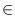Next: Straight Rays ( ray Up: Basic Data Types for Previous: Points ( point )   Contents   Index

# Segments ( segment )

Definition

An instance s of the data type segment is a directed straight line segment in the two-dimensional plane, i.e., a straight line segment [p, q] connecting two points p, qR2. p is called the source or start point and q is called the target or end point of s. The length of s is the Euclidean distance between p and q. If p = q s is called empty. We use line(s) to denote a straight line containing s. The angle between a right oriented horizontal ray and s is called the direction of s.

#include < LEDA/geo/segment.h >

Types

 segment::coord_type the coordinate type (double). segment::point_type the point type (point).

Creation

 segment s(const point& p, const point& q) introduces a variable s of type segment. s is initialized to the segment [p, q]. segment s(const point& p, const vector& v) introduces a variable s of type segment. s is initialized to the segment [p, p + v]. Precondition v.dim() = 2. segment s(double x1, double y1, double x2, double y2) introduces a variable s of type segment. s is initialized to the segment [(x1, y1),(x2, y2)]. segment s(const point& p, double alpha, double length) introduces a variable s of type segment. s is initialized to the segment with start point p, direction alpha, and length length. segment s introduces a variable s of type segment. s is initialized to the empty segment. segment s(const segment& s1, int) introduces a variable s of type segment. s is initialized to a copy of s1.

Operations

 point s.start() returns the source point of segment s. point s.end() returns the target point of segment s. double s.xcoord1() returns the x-coordinate of s.source(). double s.xcoord2() returns the x-coordinate of s.target(). double s.ycoord1() returns the y-coordinate of s.source(). double s.ycoord2() returns the y-coordinate of s.target(). double s.dx() returns the xcoord2 - xcoord1. double s.dy() returns the ycoord2 - ycoord1. double s.slope() returns the slope of s. Precondition s is not vertical. double s.sqr_length() returns the square of the length of s. double s.length() returns the length of s. vector s.to_vector() returns the vector s.target() - s.source(). double s.direction() returns the direction of s as an angle in the intervall [0, 2). double s.angle() returns s.direction(). double s.angle(const segment& t) returns the angle between s and t, i.e., t.direction() - s.direction(). bool s.is_trivial() returns true if s is trivial. bool s.is_vertical() returns true iff s is vertical. bool s.is_horizontal() returns true iff s is horizontal. int s.orientation(const point& p) computes orientation( s.source(), s.target(), p) (see below). double s.x_proj(double y) returns p.xcoord(), where pline(s) with p.ycoord() = y. Precondition s is not horizontal. double s.y_proj(double x) returns p.ycoord(), where pline(s) with p.xcoord() = x. Precondition s is not vertical. double s.y_abs() returns the y-abscissa of line(s), i.e., s.y_proj(0). Precondition s is not vertical. bool s.contains(const point& p) decides whether s contains p. bool s.intersection(const segment& t) decides whether s and t intersect in one point. bool s.intersection(const segment& t, point& p) if s and t intersect in a single point this point is assigned to p and the result is true, otherwise the result is false. bool s.intersection_of_lines(const segment& t, point& p) if line(s) and line(t) intersect in a single point this point is assigned to p and the result is true, otherwise the result is false. segment s.translate_by_angle(double alpha, double d) returns s translated in direction alpha by distance d. segment s.translate(double dx, double dy) returns s translated by vector (dx, dy). segment s.translate(const vector& v) returns s + v, i.e., s translated by vector v. Precondition v.dim() = 2. segment s + const vector& v returns s translated by vector v. segment s - const vector& v returns s translated by vector - v. segment s.perpendicular(const point& p) returns the segment perpendicular to s with source p and target on line(s). double s.distance(const point& p) returns the Euclidean distance between p and s. double s.sqr_dist(const point& p) returns the squared Euclidean distance between p and s. double s.distance() returns the Euclidean distance between (0, 0) and s. segment s.rotate(const point& q, double a) returns s rotated about point q by angle a. segment s.rotate(double alpha) returns s.rotate(s.source(), alpha). segment s.rotate90(const point& q, int i=1) returns s rotated about q by an angle of i x 90 degrees. If i > 0 the rotation is counter-clockwise otherwise it is clockwise. segment s.rotate90(int i=1) returns s.rotate90(s.source(),i). segment s.reflect(const point& p, const point& q) returns s reflected across the straight line passing through p and q. segment s.reflect(const point& p) returns s reflected across point p. segment s.reverse() returns s reversed.

Non-Member Functions

 int orientation(const segment& s, const point& p) computes orientation( s.source(), s.target(), p). int cmp_slopes(const segment& s1, const segment& s2) returns compare(slope(s1), slope(s2)). int cmp_segments_at_xcoord(const segment& s1, const segment& s2, const point& p) compares points l1v and l2v where li is the line underlying segment si and v is the vertical straight line passing through point p. bool parallel(const segment& s1, const segment& s2) returns true if s1 and s2 are parallel and false otherwise.Next: Straight Rays ( ray Up: Basic Data Types for Previous: Points ( point )   Contents   Index